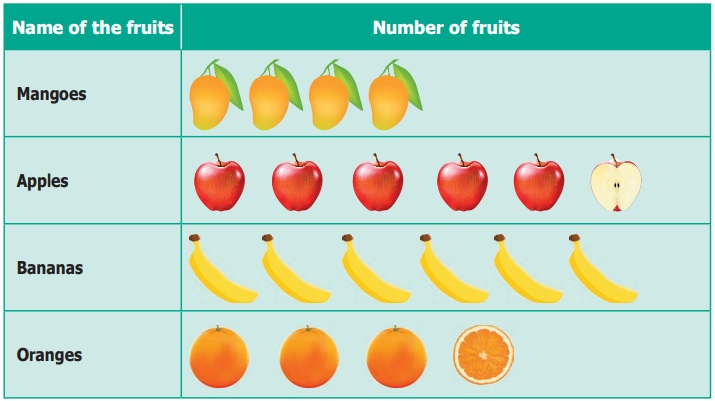Home | | Maths 6th Std | Representation of data using Pictograph

# Representation of data using Pictograph

The teacher was discussing about the pollution caused by the vehicles. The students said that they saw many vehicles when they were standing in the bus stop while coming to school.

Representation of data using Pictograph

The teacher was discussing about the pollution caused by the vehicles. The students said that they saw many vehicles when they were standing in the bus stop while coming to school. Everyone described in their own way, but Azhagi drew the pictures of the vehicles that she had seen, as shown below.All the students were able to easily understand that Azhagi had seen 5 cars, 3 lorries and 2 buses. This sort of representation of data using pictures is called Pictograph.

Nowadays pictographs are frequently used in promotion of tourism, weather forecast, geography etc.

The data can be easily analyzed and interpreted.

The pictures and symbols help us to understand better.

Note

A pictograph is the representation of data through pictures.

Do you Know?

● The pictograph is a pictorial representation for a word or Phrase.

● A pictograph is also called Pictogram.

● Pictographs were used as the earliest known form of writing examples having been discovered in Egypt and Mesopotomia since 3000 BC.

1. Need for scaling in the Pictograph

Fig. 5.4 shows, a fruit stall where there are 40 mangoes, 55 apples, 35 oranges and 60 bananas. How can we represent this data by using pictures? If the data is very large, it is very difficult and time consuming to represent each of the fruits in a pictograph. In such cases, we can use one picture to represent many of the same kind. This is called scaling.2. Drawing Pictographs

Consider the above data of fruits. 40 and 60 are multiples of 10, while 55 and 35 are multiples of 5. Let us assume, that One full picture of fruit represents 10 fruits and One half picture represents 5 fruits.

The pictograph can be drawn for the above data as shown below.3. Interpreting pictograph

From the above pictograph the number of fruits can be calculated very easily.

Number of Mangoes = 4 full pictures 4 × 10 = 40 mangoes

Number of Apples = 5 full pictures and 1 half picture (5 × 10) + 5 = 55 apples

Number of Bananas = 6 full pictures 6 × 10 = 60 bananas

Number of Oranges = 3 full pictures and 1 half picture (3 × 10) + 5 = 35 oranges

Example 5.2

The following table shows the number of vehicles sold in a year.Look at the pictograph and answer the following questions.

(i) How many motor cycles were sold in a year?

(ii) Number of buses sold in a year is 20. Say True or False.

(iii) How many bicycles were sold ?

(iv) How many cars and vans were sold?

(v) How many vehicles were sold altogether?

Solution

Given : 1 picture represents 10 vehicles

(i) There were 9 × 10 = 90 motor cycles sold.

(ii) True.

(iii) There were 4 × 10 = 40 bicycles sold.

(iv) There were 7 cars and 3 vans pictures.

Therefore 70 + 30 = 100 cars and vans sold.

(v) There were 7 cars, 3 vans, 9 motor cycles, 2 buses and 4 bicycles sold. Therefore, 70 + 30 + 90 + 20 + 40 = 250 vehicles sold.

Example 5.3

The pictograph shows the number of branded mobile phones sold in five months.Observe the given table and answer the following questions.

(i) In which month was the maximum number of brand B mobiles sold?

(ii) In which month was equal number of brand A and brand B mobiles sold?

(iii) In which month was the minimum number of brand A mobiles sold?

(iv) Find the total number of Brand A Mobiles that were sold in 5 months.

(v) What is the difference between the sale of brand A and brand B Mobiles in the month of May?

Solution

(i) February

(ii) April

(iii) March

(iv) 1250

(v) Brand A has sold 200 mobiles more than brand B

Activity

Collect from class VI students data regarding games they like and then draw a pictograph for the data collected.

Tags : Statistics | Term 1 Chapter 5 | 6th Maths , 6th Maths : Term 1 Unit 5 : Statistics
Study Material, Lecturing Notes, Assignment, Reference, Wiki description explanation, brief detail
6th Maths : Term 1 Unit 5 : Statistics : Representation of data using Pictograph | Statistics | Term 1 Chapter 5 | 6th Maths